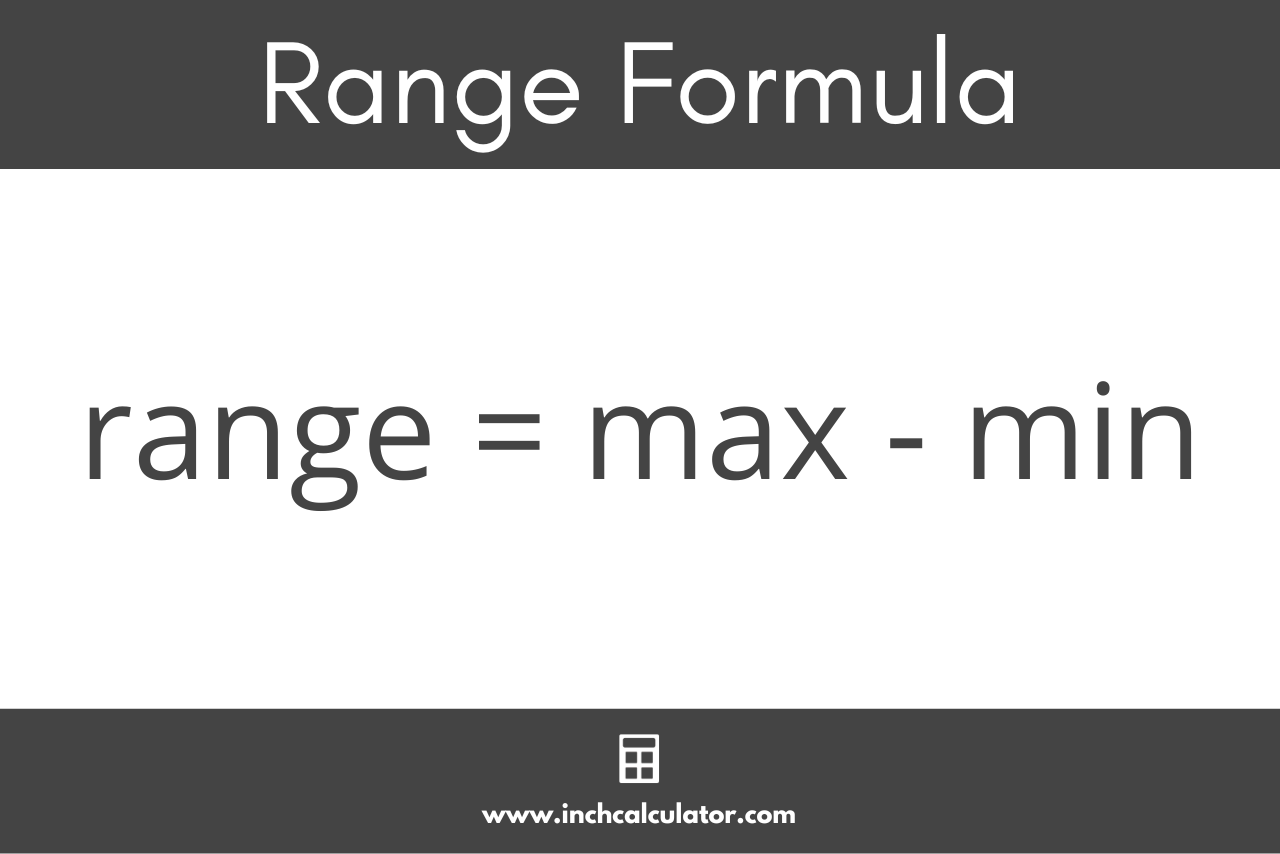# Range Calculator

Find the range for a set of data by entering the numbers in the data set below. Read below to learn more about how to find the range.

Separate numbers using a comma (,)

## Results:

### Steps to Solve

Min = ?

Max = ?

#### Step Two: Find the Range

Range = Max - Min

Learn how we calculated this below

## How to Find Range

In statistics, the range is the difference between the smallest and largest numbers in a data set. The range is used to measure the spread or dispersion between numbers in a set of values.

The range is closely related to the midrange, which is a measure of center, or central tendency, of the data.

A very large range indicates that the data might be spread out or that there are outliers, while a small range means the data are close together.

### Range Formula

Since the range is the difference between the minimum and maximum numbers in the data, the range formula is:

range = max – min

Where:
max = highest number
min = lowest number

Thus, the range is equal to the maximum value in the data minus the minimum value.The first step to find the range is to identify the smallest and largest numbers in the data. We’ll refer to these as the min and max in formulas.

Then, you can subtract the smallest from the largest to find the range.

For example: let’s find the range for the numbers [2,12,43,72]

min = 2
max = 72
range = 72 – 2 = 70

In this example, the range is 70, which is a large spread between the highest and lowest numbers.

Next, let’s find the range for the numbers [19,20,22,23]

min = 19
max = 23
range = 23 – 19 = 4

The range in this example is 4, which is a considerably smaller spread than the previous example.

You might also be interested in our mean, median, mode calculator to find additional measurements about your data.